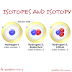Isotopy and isotopes

Isotopy can be defined as a process/occurrence/phenomenon whereby an element has the same atomic number (i.e the number of protons) but different mass number (i.e number of neutrons).

• Proton
• Neutron
• Electron

Isotopes are the elements that have the same atomic number (number of protons) and different mass number (number of neutrons). Examples of isotopes are;

Chlorine    3517Cl , 3717Cl

Oxygen        168O , 178O , 188O

Hydrogen     11H ,21 H , 31H

Carbon          126C , 136C

Sodium      2311Na , 2411Na

Lithium           63Li  , 73Li

Worked Examples:
Question 1
there are two isotopes of chlorine with mass numbers 35 and 37 respectively. if the isotopes exist in the ratio 3:1 , the lighter isotope being more abundant. what is the relative atomic mass of chlorine?

solution
1st isotope   35Cl
2nd isotope   37Cl
the isotopic ratio = 3:1
Total ratio = 3 + 1 = 4
% abundance of  35Cl = (3/4 x 100) = 75%
% abundance of  37Cl = (1/4 x 100) = 25%
Relative atomic mass of chlorine (R.A.M) = (75/100 X 35) + (25/100 X 37) = 3550/100
Relative atomic mass of chlorine (R.A.M) = 35.5

Question 2
Boron exist as an isotopic mixture containing 80% of B , 15% of B and 5% of B. Calculate the  relative atomic mass of Boron?

solution
1st isotope   9B
2nd isotope 10B
3rd isotope 11B
the % abundance ratio = 80:15:5

Relative atomic mass of chlorine (R.A.M) = (80/100 X 9) + (15/100 X 10) +  (5/100 X 11)  = 925/100
Relative atomic mass of boron (R.A.M) = 92.5

Question 3
If the relative atomic mass of natural copper is 63.54. Calculate the proportions of isotopes 63CU and 65CU in the metal?

solution
1st isotope   63Cu
2nd isotope   65 Cu
Let the proportion of cu be x
Let the proportion of cu be y
therefore, x + y = 100  ...................(1)
Relative atomic mass of copper (R.A.M) = (x/100 X 63) + (y/100 X 65) = 63.54
63.54 = 0.63x + 0.65y   .............(2)
from equation (1);  y = 100 - x
substitute for y in the equation (2) above  63.54 = 0.63x + 0.65(100 - x)
63.54 = 0.63x + 65 - 0.65x
1.46 = 0.02x
x = 0.73 (=73%)
recall; y = 100 - x
y = 100 - 73
y = 27%

Question 4
Two isotopes of z with mass number 18 and 20 are in the ratio 1:2. Determine the relative atomic mass.

solution
1st isotope   20Z
2nd isotope  18 Z
the isotopic ratio = 1:2
Total ratio = 2 + 1 = 3
% abundance of  20 Z = (2/3 x 100) = 66.6%
% abundance of  18 Z = (1/3 x 100) = 33.3%
Relative atomic mass of Z (R.A.M) = (66.6/100 X 20) + (33.3/100 X 18) = 1931.4/100
Relative atomic mass of Z (R.A.M) = 19.314.

Isotopy and Isotopes; definition,questions and solution. - chemistryReviewed by uyi osamudiamen Lucky on August 16, 2019 Rating: 5

#### 1 comment:

1.